Thermochemistry 2012 VCE 1) The diagram below represents the distribution of the kinetic energy of reactant particles at two different temperatures. Assume that the areas under the curves are equal.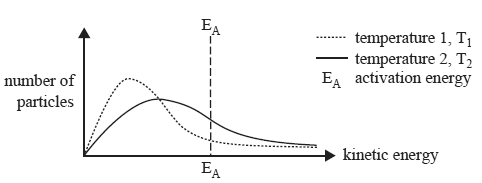From this diagram it can be concluded that a) at T1 a greater number of particles have sufficient energy to react. T1 is greater than T2. b) at T1 a greater number of particles have sufficient energy to react. T2 is greater than T1. c) at T2 a greater number of particles have sufficient energy to react. T1 is greater than T2. d) at T2 a greater number of particles have sufficient energy to react. T2 is greater than T1. Solution2) Enthalpy changes for the melting of iodine, I2, and for the sublimation of iodine are provided below.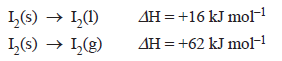The enthalpy change for the vaporisation of iodine that is represented by the equation I2(l) => I2(g) is A. –78 kJ mol–1 B. –46 kJ mol–1 C. +46 kJ mol–1 D. +78 kJ mol–1 Solution 3) Nitrogen dioxide decomposes as follows.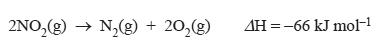The enthalpy change for the reaction represented by the equation below is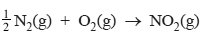A. –66 kJ mol–1 B. –33 kJ mol–1 C. +33 kJ mol–1 D. +66 kJ mol–1 Solution4) Pure water at 100 °C has a pH of 6.14. This is because a) the self-ionisation of water is endothermic. b) pH measurements at this temperature are unreliable. c) pH measurements are affected by the bubbles of hydrogen gas that form in boiling water. d) the concentration of H3O+ ions is not equal to the concentration of OH– ions at this temperature. Solution5) Consider the following energy profi le diagram for a reaction represented by the equation X + Y => Z.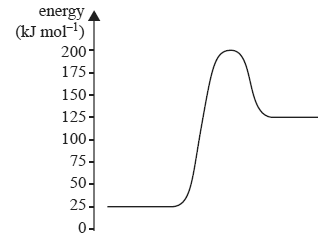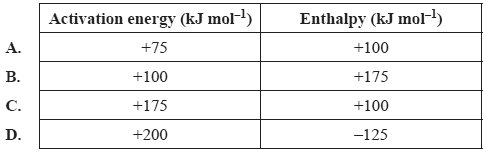Solution6) 1.30 g of glucose (M = 180 g mol–1) underwent complete combustion. The energy released was used to heat an unknown mass of water. If the temperature of the water increased by 24.3 °C and it is assumed no heat was lost, the mass of the water heated was a) 2.00 × 10–1 g b) 1.02 × 102 g c) 2.00 × 102 g d) 3.84 × 103 g Solution7) When 50 g of water at 90 °C is added to a calorimeter containing 50 g of water at 15 °C, the temperature increases to 45 °C. Assuming no energy is lost to the environment, the energy absorbed by the calorimeter is equal to the a) energy lost by the hot water. b) energy gained by the cold water. c) sum of the energy gained by the cold water and the energy lost by the hot water. d) difference between the energy lost by the hot water and the energy gained by the cold water. Solution 8) If 54.0 kJ of energy is required to convert 1.00 mol of liquid water to steam at 100 °C, the amount of heat energy, in kilojoule, required to convert 100 g of water at 20 °C to steam at 100°C is A. 3.34 × 101 kJ B. 2.67 × 102 kJ C. 3.00 × 102 kJ D. 3.33 × 102 kJ Solution 9) The reaction between 2-bromo-2-methylpropane and hydroxide ions occurs in two steps.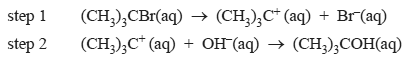a) Write an equation that represents the overall reaction between 2-bromo-2-methylpropane and hydroxide ions. Solutionb) The energy profile diagrams for step 1 and step 2 are shown below. Both are drawn to the same scale.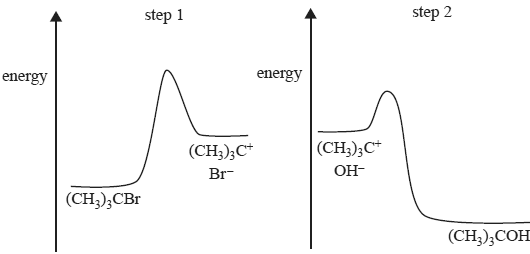i. Which step involves an endothermic reaction? Provide a reason for your answer. Solution The reaction at step 1 occurs at a different rate to the reaction at step 2. ii. Which step is slower? Justify your answer. Solution10) Methanol, CH3OH, undergoes combustion according to the equation 2CH3OH(l) + 3O2(g) => 2CO2(g) + 4H2O(g) In an experiment to determine its suitability as a fuel, a sample of methanol underwent complete oxidation in a bomb calorimeter. The calorimeter was first calibrated by passing a current through an electric heater placed in the water surrounding the reaction vessel. A potential of 5.25 volts was applied for 3.00 minutes. The measured current was 1.50 amperes and the temperature of the water and reaction vessel increased by 0.593 °C. a) Determine the calibration constant, in kJ °C–1, for the calorimeter and its contents. Solutionb) A student then used this calorimeter to determine the molar heat of combustion of methanol. 0.934 g of methanol was placed in the reaction vessel and excess oxygen was added. An electric ignition heater provided the energy required to initiate the combustion reaction. On this occasion, the temperature of the water increased by 8.63 °C. Use this experimental data to determine the value of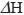for the combustion of methanol given by the following equation. 2CH3OH(l) + 3O2(g) => 2CO2(g) + 4H2O(g) Include appropriate units in your answer. Solution c) The value of, calculated using the enthalpy of combustion provided in the data book, is different from the value ofcalculated from the experimental data provided in part b). Provide a reason for this difference.   Solution